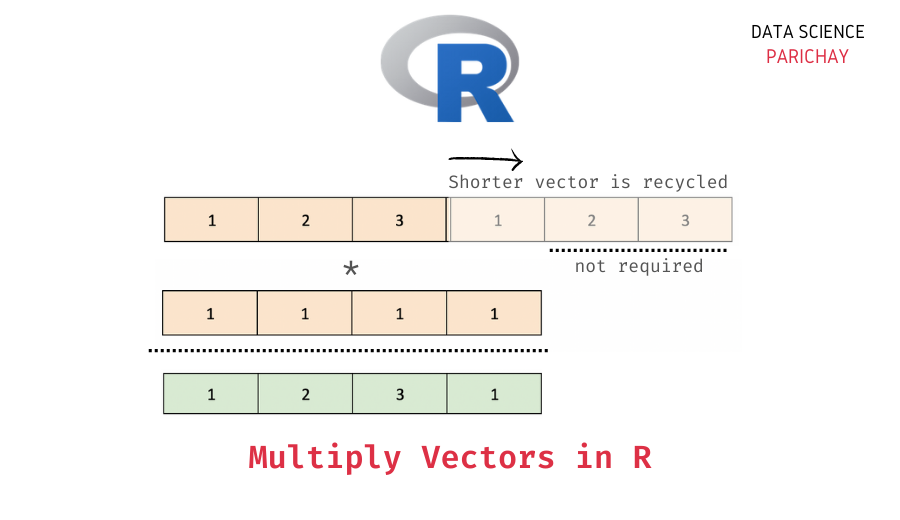# vector## Common Trigonometric Functions in R

In this tutorial, we will look at the common trigonometric functions in the R programming language with the help of some examples. How to compute the sine, cosine, and tan of a value in R? R comes with built-in methods for common trigonometric functions such as sine, cosine, and tan. The following is the syntax …## R – Multiply Two Vectors Elementwise

In this tutorial, we will look at how to multiply two vectors (element-wise) in the R programming language with the help of some examples. Elementwise Vector Multiplication in R You can use the * operator to multiply two vectors in R. Arithmetic operations on vectors are computed element-wise. That is when you multiply two vectors, …

Scroll to Top